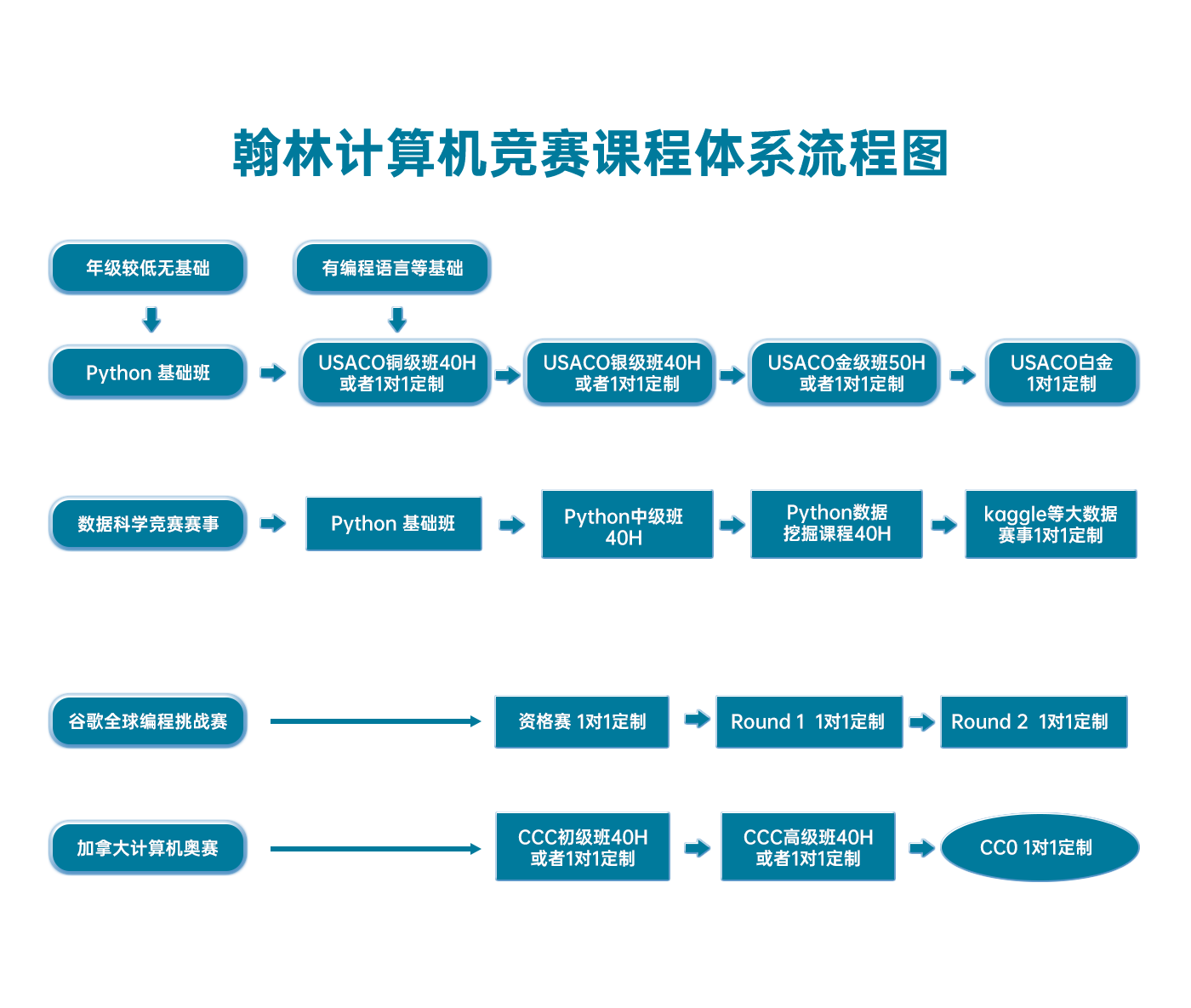# USACO 2015 January Contest, Gold Problem 2. Moovie Mooving

USACO2015JAN-G2

(Analysis by Steven Hao)

Because the bounds on N are small, a solution with exponential runtime will be fast enough. Following this train of thought, we naturally come upon a dynamic programming solution with 2N states: the subsets of {0,1,2,...,N1}{0,1,2,...,N−1}.

For every set of movies M, we compute the maximum amount of time Bessie can stay safe watching movies only from M. Then, the final answer is the minimum size of any set which keeps Bessie safe for at least minutes.

The transiition between states is fairly simple. If Bessie has already watched movies from set M, and wishes to watch another movie i, then the new state will beM∪{i}. To maximize the amount of time she stays safe, Bessie should catch the latest showing of i possible.

This yields a recurrence: where

• DPM is the maximum amount of time spent watching movies from MM
• Di is the length of movie i
• Si is the set of showtimes of movie i

the amount of time Bessie is safe watching SS then ii is given by

t=max(sSisDPM)+Dit=max(s∈Si∣s≤DPM)+Di

Where M=MiM′=M∪i, we may then update DPMDPM′ as:

DPM=max(DPM,t)DPM′=max(DPM′,t)

The slowest step is in computing t; we binary search for the insertion point of DPMDPM in SiSi. Where CC is the number of showtimes per movie, this transition can be computed in O(logC)O(log⁡C) time.

As there are 2N2N states and O(N)O(N) transitions from each state, this yields an overall runtime of O(2NNlogC)O(2NNlog⁡C), which is barely fast enough.

If it is not fast enough, the searching step can be removed and replaced with a bit of precomputation: For all cciijj: compute the latest showing of j you can watch after watching the cc-th showing of movie. This takes O(CN2)O(CN2) precomputation, and yields a runtime of O((2N+CN)N)O((2N+CN)N). However, implementing this one requires storing not just the maximum safe time but the movie and showtime that yields it.

Below is my implementation of the O(2NNlogC)O(2NNlog⁡C) solution.

#include <cstdio>

const int MAXN = 22;
const int MAXC = 1010;

int N, L;

int D[MAXN];        // D[i]: length of movie i
int S[MAXN][MAXC]; // S[i]: showtimes of movie i
int C[MAXN];        // C[i]: length of list ar[i]

int dp[1 << MAXN]; // dp[ {x} ]: max safe time watching movies from {x}
// where {x} is a subset of [0,N), and is represented by a bitmask

int find(int val, int *ar, int len) {
// bin search for greatest x s.t. ar[x] <= val
int lo = -1, hi = len - 1;
while (lo < hi) {
int mid = (lo + hi + 1) / 2;
if (ar[mid] <= val) {
lo = mid;
} else {
hi = mid - 1;
}
}
return lo;
}

int popcount(int x) { // returns number of bits of x set to 1
if (x) return 1 + popcount(x & (x - 1));
else return 0;
}

int main() {
if (fopen("movie.in", "r")) {
freopen("movie.in", "r", stdin);
freopen("movie.out", "w", stdout);
}

scanf("%d %d", &N, &L);
for(int i = 0; i < N; ++i) {
scanf("%d %d", D + i, C + i);
for(int j = 0; j < C[i]; ++j) {
scanf("%d", S[i] + j);
}
}

for(int msk = 1; msk < (1 << N); ++msk) {
dp[msk] = -1;
}
// dp = 0

int ans = -1;
for(int msk = 0; msk < (1 << N); ++msk) {
int cur = dp[msk];
if (cur == -1) continue;

if (cur >= L) {
int cnt = popcount(msk);
if (ans == -1 || cnt < ans) ans = cnt;
}

for(int i = 0; i < N; ++i) {
if (msk & (1 << i)) continue;
// try watching movie i after watching {msk}
int nmsk = msk | (1 << i);
int idx = find(cur, S[i], C[i]);
if (idx == -1) continue;
// want to watch idx-th showing of movie i (latest showing)

int t = S[i][idx] + D[i];
if (t > dp[nmsk]) dp[nmsk] = t;
}
}

printf("%d\n", ans);
return 0;
}

### 翰林USACO课程体系流程图